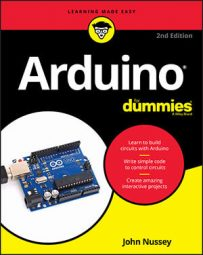##### Arduino For DummiesWhen building your Arduino projects, you use resistors to limit the amount of current going to certain components in the circuit, such as LEDs and integrated circuits. To calculate the resistance, you should use a modified version of Ohm’s Law.

In the following equation, R is resistance; VSUPPLY is the voltage supplied from the power source (this is 5V for a standard Arduino digital pin, but could be more or less if the VIN pin is used); VFORWARD is the voltage required by the component, and I is the current required by the component:

R = (VSUPPLY - VFORWARD) / I

Here is an example for powering an LED:

(5V – 2V) / 0.03A = 100Ω

After you’ve determined which resistor you need, the next task is to find it. Fixed-value resistors use colored bands to indicate the value of the resistor. To find the value you can use a multimeter on the ohms (Ω) setting, but if you don’t have a multimeter handy, use the following table to find the value instead. For example, a resistor with brown, black, brown, and gold bands is a 100Ω resistor with a 5% tolerance.

In addition, the Circuit Playground by Adafruit has resistor color codes as well as a variety of other useful tools for building circuits.

Resistor Color Chart
Color Value Multiplier Tolerance
Black 0 x100 -
Brown 1 x101 ±1%
Red 2 x102 ±2%
Orange 3 x103 -
Yellow 4 x104 ±5%
Green 5 x105 ±0.5%
Blue 6 x106 ±0.25%
Violet 7 x107 ±0.1%
Grey 8 x108 ±0.05%
White 9 x109 -
Gold - x10-1 ±5%
Silver - x10-2 ±10%
None - - ±20%